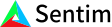1. Bob Anderson
×
IntuitiveML

## What is the difference between a Deep Neural Network and an Artificial Neural Network?

Jun 1, 2020

Technically, an artificial neural network (ANN) that has a lot of layers is a Deep Neural Network (DNN). In practice though, a deep neural network is just a normal neural network where the layers of the network are abstracted out, or a network that uses functions not typically found in an artificial neural network. For example, you could draw an artificial neural network like this. Then, you could abstract this as an input, 3 fully connected layers, and an output. You could then reasonably say that this is a deep neural network. For most real-life applications, you would want more layers or at least more nodes per layer than what I’ve drawn here, but that’s the idea. Additionally, a fully connected layer is just a normal layer of an artificial neural network, where the layer is made up of neurons, each of which take all the previous layer’s neurons as input.

A deep neural network doesn’t have to be an artificial neural network though. In a deep neural network, you can use whatever formulas and techniques you want. For instance, a deep neural network could have three fully connected blocks attached to the input, then take the hyperbolic tangent of the output of the first block and the sigmoid of the second. Then sum these two blocks together before multiplying that by the third block. In a deep neural network, you can use whatever techniques or mathematical formulas you want to shape each layer or section of your network, but in artificial neural networks you are limited to just using fully connected layers.